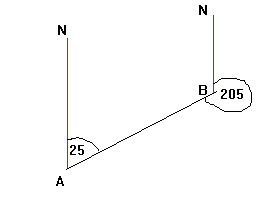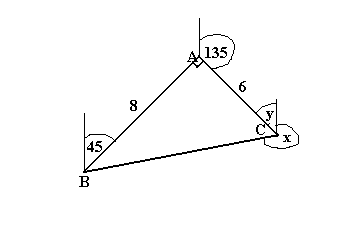## Bearings

A bearing is an angle, measured clockwise from the north direction. Below, the bearing of B from A is 025 degrees (note 3 figures are always given). The bearing of A from B is 205 degrees.Example

A, B and C are three ships. The bearing of A from B is 045º. The bearing of C from A is 135º. If AB= 8km and AC= 6km, what is the bearing of B from C?tanC = 8/6, so C = 53.13º
y = 180º - 135º = 45º (interior angles)
x = 360º - 53.13º - 45º (angles round a point)
= 262º (to the nearest whole number)

This video shows you how to work out Bearings questions.